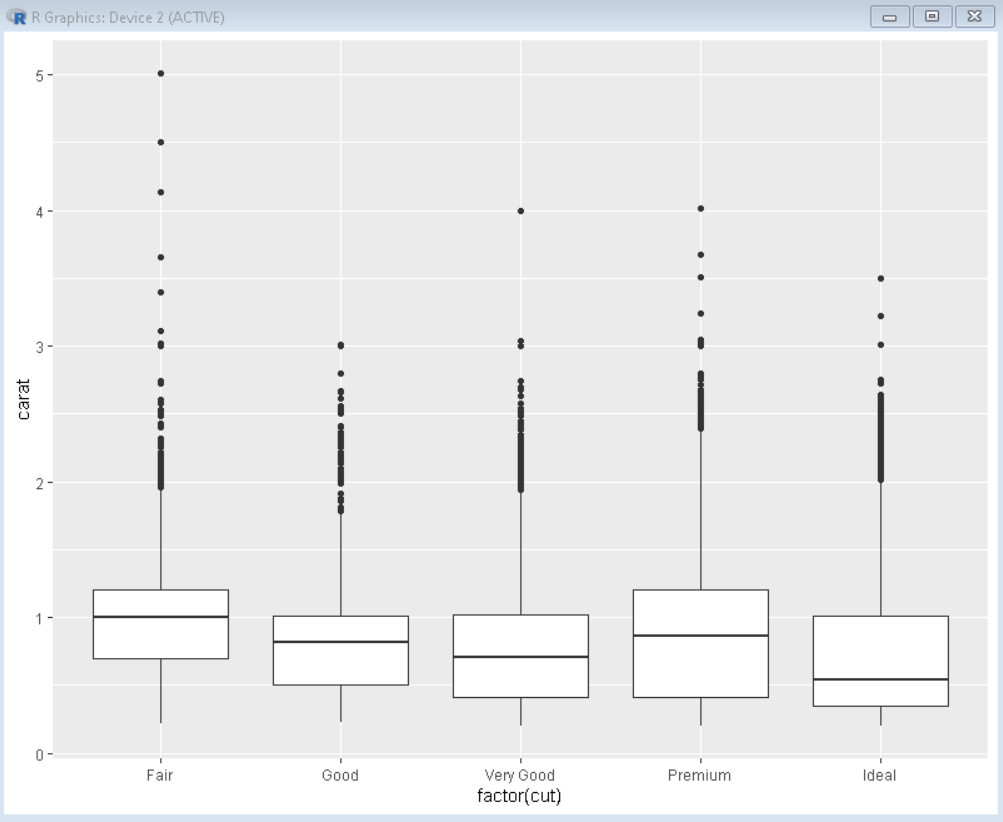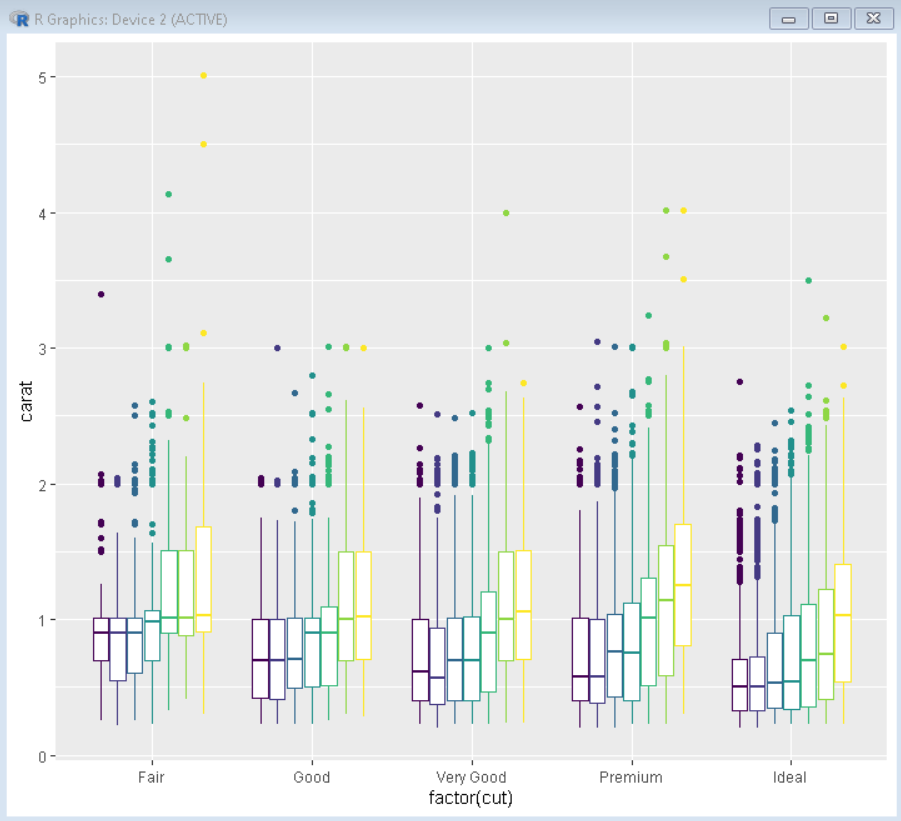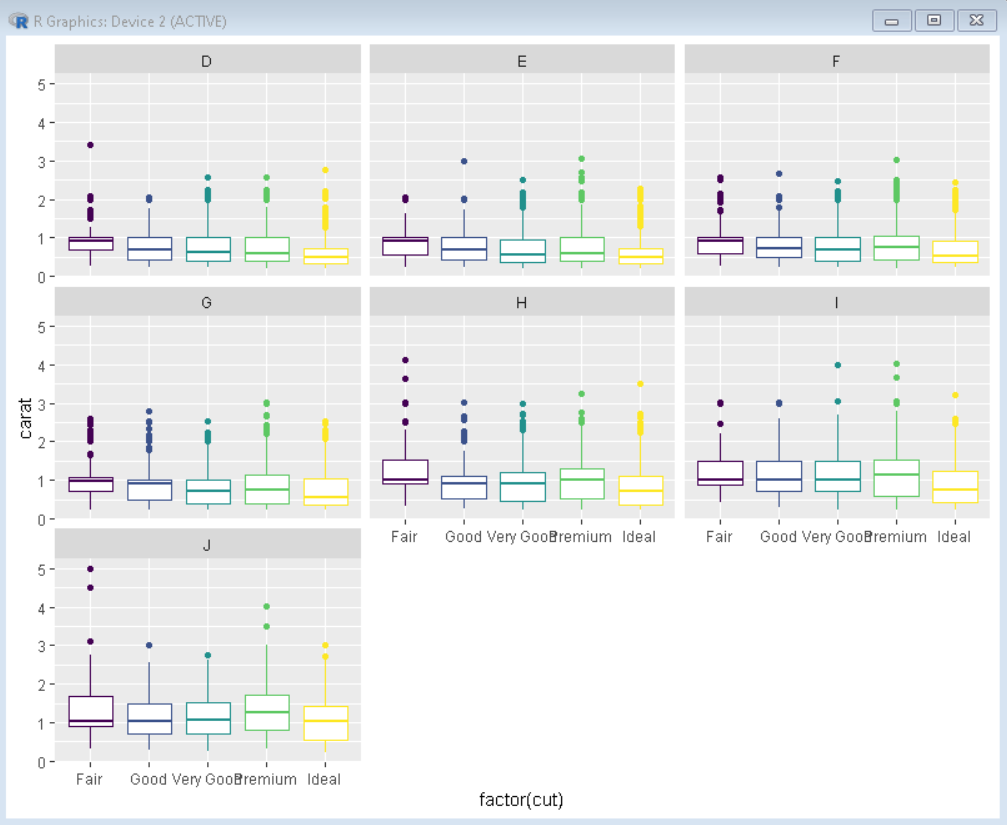Open in App
Not now

# How to Make Grouped Boxplots with ggplot2 in R?

• Last Updated : 03 Dec, 2021

In this article, we will discuss how to make a grouped boxplot in the R Programming Language using the ggplot2 package.

Boxplot helps us to visualize the distribution of quantitative data comparing different continuous or categorical variables. Boxplots consist of a five-number summary which helps in detecting and removing outliers from the dataset. These five summary numbers are Minimum, First Quartile, Median, Third Quartile, and Maximum.

Grouped Boxplots are used to visualize the data having multiple subgroups. Also, we can visualize three variables at a time with grouped boxplots where one variable is numerical and the other two are categorical variables. We can visualize the fourth variable by using the color property of ggplot in R.

First, to make a basic boxplot in R using the ggplot2 package, we use the geom_boxplot() function in the R Language.

Syntax:

ggplot(dataframe,  aes( x, y ) ) + geom_boxplot()

Example:

Here, is a basic boxplot made using the geom_boxplot function of the ggplot2 package.

## R

 `# load library ggplot``library``(ggplot2)`` ` `# Plot boxplot using ggplot function``# diamonds dataset used here is inbuilt in the R Language``plot <- ``ggplot``(diamonds, ``aes``(x=``factor``(cut), y=carat))+``     ``geom_boxplot``()+``     ``theme``( legend.position = ``"none"` `)` `# print boxplot``plot`

Output:## Creating grouped boxplot

To convert this basic boxplot to grouped boxplot we use the color property of aes() function of the ggplot2 package. Here, we use a third variable as the value of argument color to convert a basic boxplot to grouped boxplot.

Syntax:

ggplot(dataframe,  aes( x, y, color = z ) ) + geom_boxplot()

Parameters:

• x is first categorical variable
• y is quantitative variable
• z is second categorical variable.

Example:

Here, is a boxplot grouped by variable color in ggplot2. The diamonds dataset used in example is pre-built in R Language.

## R

 `# load library ggplot``library``(ggplot2)`` ` `# Plot boxplot using ggplot function``# diamonds dataset used here is inbuilt in the R Language``plot <- ``ggplot``(diamonds, ``aes``(x=``factor``(cut), y=carat, color = ``factor``(color)))+``     ``geom_boxplot``()+``     ``theme``( legend.position = ``"none"` `)` `# print boxplot``plot`

Output:## Grouped Boxplots with facets

There is one more way to make a grouped boxplot in the R language using the ggplot2 package. It is to use facet in ggplot. The faceting functions in ggplot2 offer a general solution to split up the data by one or more variables and make plots with subsets of data together. To create a grouped boxplot, we can use the facet_wrap() function.

Syntax:

ggplot(dataframe,  aes( x, y ) ) + geom_boxplot() + facet_wrap(~z)

Parameters:

• x is first categorical variable
• y is quantitative variable
• z is second categorical variable

Example:

Here, is a boxplot grouped by variable color in ggplot2 using facet_wrap() function. The diamonds dataset used in the example is pre-built in R Language. We have also colored the plot by using the cut variable as a value to color argument in the ggplot() function.

## R

 `# load library ggplot``library``(ggplot2)`` ` `# Plot boxplot using ggplot function``# diamonds dataset used here is inbuilt in the R Language``plot <- ``ggplot``(diamonds, ``aes``(x=``factor``(cut), y=carat, color=``factor``(cut) ))+``     ``geom_boxplot``()+``     ``theme``( legend.position = ``"none"` `)+``     ``facet_wrap``(~color, ncol=3)` `# print boxplot``plot`

Output:My Personal Notes arrow_drop_up